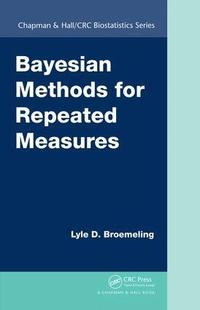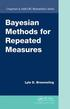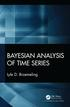Fler böcker inom
Format
Inbunden (Hardback)
Språk
Engelska
Antal sidor
568
Utgivningsdatum
2015-08-17
Förlag
Illustrationer
4227 total equations; 221 Tables, black and white; 86 Illustrations, black and white
Dimensioner
236 x 152 x 33 mm
Vikt
931 g
Antal komponenter
1
ISBN
9781482248197

### Du kanske gillar# Bayesian Methods for Repeated Measures

Inbunden Engelska, 2015-08-17
1359
Skickas inom 7-10 vardagar.
Fri frakt inom Sverige för privatpersoner.
Finns även som
579
• Skickas inom 10-15 vardagar
739
Analyze Repeated Measures Studies Using Bayesian Techniques Going beyond standard non-Bayesian books, Bayesian Methods for Repeated Measures presents the main ideas for the analysis of repeated measures and associated designs from a Bayesian viewpoint. It describes many inferential methods for analyzing repeated measures in various scientific areas, especially biostatistics. The author takes a practical approach to the analysis of repeated measures. He bases all the computing and analysis on the WinBUGS package, which provides readers with a platform that efficiently uses prior information. The book includes the WinBUGS code needed to implement posterior analysis and offers the code for download online. Accessible to both graduate students in statistics and consulting statisticians, the book introduces Bayesian regression techniques, preliminary concepts and techniques fundamental to the analysis of repeated measures, and the most important topic for repeated measures studies: linear models. It presents an in-depth explanation of estimating the mean profile for repeated measures studies, discusses choosing and estimating the covariance structure of the response, and expands the representation of a repeated measure to general mixed linear models. The author also explains the Bayesian analysis of categorical response data in a repeated measures study, Bayesian analysis for repeated measures when the mean profile is nonlinear, and a Bayesian approach to missing values in the response variable.

## Passar bra ihop

1.2. +
3.De som köpt den här boken har ofta också köpt Bayesian Analysis of Time Series av Lyle D Broemeling (inbunden).

## Kundrecensioner

Har du läst boken? Sätt ditt betyg »

## Recensioner i media

"The book treats these topics from a Bayesian perspective using WinBugs as the software of choice. The WinBugs code is available on a website and can be used as the reader progresses through the book. The worked examples are often from biostatistics. The intended audience is "graduate students in statistics (including biostatistics) and as a reference for consulting statisticians."While there are other excellent books on Repeated Measures models, this book is unique in adopting a Bayesian perspective. The book is comprehensive." ~David E. Booth, Kent State University "The book will be especially useful for clinical researchers, epidemiologists, and other researchers focused on data analysis and seeking to apply Bayesian methods. Useful computer codes and worked examples are provided. Moreover, the book also has utility as a general exposition of data and graph analytic approaches to longitudinal data." ~Peter Congdon, Biometric Journal

## Övrig information

Lyle D. Broemeling has 30 years of experience as a biostatistician. He has been a professor at the University of Texas Medical Branch at Galveston, the University of Texas School of Public Health at Houston, and the University of Texas MD Anderson Cancer Center. He is also the author of several books, including Bayesian Methods in Epidemiology. His research interests include the analysis of repeated measures and Bayesian methods for assessing medical test accuracy and inter-rater agreement.

## Innehållsförteckning

Introduction to the Analysis of Repeated Measures Introduction Bayesian Inference Bayes's Theorem Prior Information Posterior Information Posterior Inference Estimation Testing Hypotheses Predictive Inference The Binomial Forecasting from a Normal Population Checking Model Assumptions Sampling from an Exponential, but Assuming a Normal Population Poisson Population Measuring Tumor Size Testing the Multinomial Assumption Computing Example of a Cross-Sectional Study Markov Chain Monte Carlo Metropolis Algorithm Gibbs Sampling Common Mean of Normal Populations An Example Additional Comments about Bayesian Inference WinBUGS Preview Exercises Review of Bayesian Regression Methods Introduction Logistic Regression Linear Regression Models Weighted Regression Nonlinear Regression Repeated Measures Model Remarks about Review of Regression Exercises Foundation and Preliminary Concepts Introduction An Example Notation Descriptive Statistics Graphics Sources of Variation Bayesian Inference Summary Statistics Another Example Basic Ideas for Categorical Variables Summary Exercises Linear Models for Repeated Measures and Bayesian Inference Introduction Notation for Linear Models Modeling the Mean Modeling the Covariance Matrix Historical Approaches Bayesian Inference Another Example Summary and Conclusions Exercises Estimating the Mean Profile of Repeated Measures Introduction Polynomials for Fitting the Mean Profile Modeling the Mean Profile for Discrete Observations Examples Conclusions and Summary Exercises Correlation Patterns for Repeated Measures Introduction Patterns for Correlation Matrices Choosing a Pattern for the Covariance Matrix More Examples Comments and Conclusions Exercises General Mixed Linear Model Introduction and Definition of the Model Interpretation of the Model General Linear Mixed Model Notation Pattern of the Covariance Matrix Bayesian Approach Examples Diagnostic Procedures for Repeated Measures Comments and Conclusions Exercises Repeated Measures for Categorical Data Introduction to the Bayesian Analysis with a Dirichlet Posterior Distribution Bayesian GEE Generalized Mixed Linear Models for Categorical Data Comments and Conclusions Exercises Nonlinear Models and Repeated Measures Nonlinear Models and a Continuous Response Nonlinear Repeated Measures with Categorical Data Comments and Conclusion Exercises Bayesian Techniques for Missing Data Introduction Missing Data and Linear Models of Repeated Measures Missing Data and Categorical Repeated Measures Comments and Conclusions Exercises References